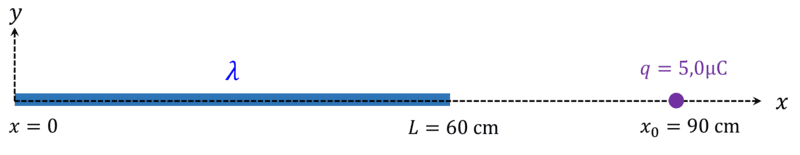# Force on a particle of a linear charge distribution

Guillem_dlc
Thread moved from the technical forums to the schoolwork forums
Hello!

I am trying to solve this exercise of the electric field, but it comes out changed sign and I don't know why.

Statement: On a straight line of length ##L=60\, \textrm{cm}## a charge ##Q=3,0\, \mu \textrm{C}## is uniformly distributed. Calculate the force this linear distribution makes on a point charge ##q=5,0\, \mu \textrm{C}## in the same direction of the thread and at a distance of ##30\, \textrm{cm}## from one of its ends.My solution: First, we want to look at how much the electric field is worth at different points. We choose an infinitesimal charge ##dq## any at any point in the thread ##(x,0)##, and we assign to the point charge any ##(x_0,0)## that fulfils the condition ##x_0>L##. According to this, we can already define the vector ##\overrightarrow{r}## that arises in the charge differential and ends at point ##(x_0,0)##:
$$\overrightarrow{r}=(x_0,0)-(x,0)=(x_0-x,0)$$
$$r=x_0-x\rightarrow \widehat{r}=\dfrac{\overrightarrow{r}}{r}=(1,0)$$
$$\lambda =\dfrac{dq}{dl}\rightarrow dq=\lambda dl$$
where ##dl=dx## because we only have component ##x##.
$$E=\int_L k\dfrac{dq}{r^2}\widehat{r}=k\int_0^L \dfrac{\lambda \, dx}{(x_0-x)^2}(1,0)$$
Then,
$$E=k\lambda \widehat{i}\int_0^L \dfrac{1}{(x_0-x)^2}\, dx = k\lambda \widehat{i} \left[ \boxed{\dfrac{-1}{(x_0-x)}}\right]_0^L = k\lambda \widehat{i} \left( \dfrac{-1}{x_0-L}+\dfrac{1}{x_0}\right)$$
$$=\dfrac{-k\lambda}{x_0-L}+\dfrac{k\lambda}{x_0}=\dfrac{-k\lambda}{0,3}+\dfrac{k\lambda}{0,9}=\dfrac{-3k\lambda +k\lambda}{0,9}=$$
$$=\dfrac{-2k\lambda}{0,9}=-100000\, \textrm{V}/\textrm{m},$$
using that ##\lambda =\dfrac{dq}{dL}=\dfrac{Q}{L}=0,000005##. Finally,
$$F=qE=-0,5\, \textrm{N}$$

My question: It gives me good, but it's changed in sign and I think that's why I've marked in the integral, but I'd say I've done it well...

Homework Helper
Gold Member
$$E=k\lambda \widehat{i}\int_0^L \dfrac{1}{(x_0-x)^2}\, dx = k\lambda \widehat{i} \left[ \boxed{\dfrac{-1}{(x_0-x)}}\right]_0^L$$

You are right to suspect that the problem is with the evaluation of the integral. You got $$\dfrac{-1}{(x_0-x)}$$ for an antiderivative of $$\dfrac{1}{(x_0-x)^2}$$

Does the derivative of ##\dfrac{-1}{(x_0-x)}## with respect to ##x## equal ##\dfrac{1}{(x_0-x)^2}##?

Last edited:
•vanhees71